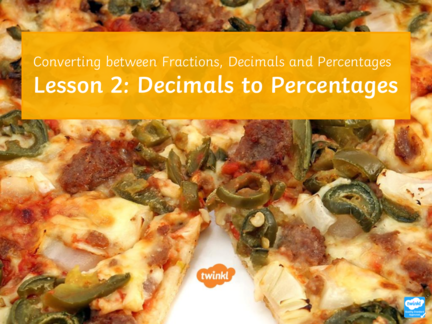# Decimals to PercentagesContributed by:From this pdf, students will learn to develop an understanding of how to convert and write equivalent decimals and percentages and also:
1. To multiply and divide by 100.
2. To convert decimals to percentages.
3. To convert percentages to decimals.
1. Converting between Fractions, Decimals and Percentages
Lesson 2: Decimals to Percentages
2. Learning Objective
To develop an understanding of how to convert and write equivalent
decimals and percentages.
Targeting Assessment Objective A01
Success Criteria
• To multiply and divide by 100.
• To convert decimals to percentages.
• To convert percentages to decimals.
3. Quick Maths
Calculate each of the following without a calculator.
1. 45 × 1000 = 45 000 1. 95 ÷ 10 = 9.5 1. 82 ÷ 100 = 0.82
2. 990 × 10 = 9900 2. 485 × 100 = 48 500 2. 79.2 ÷ 1000 = 0.0792
3. 550 ÷ 10 = 55 3. 6.2 × 10 = 62 3. 0.48 × 10 = 4.8
4. 90 ÷ 10 = 9 4. 0.52 × 1000 = 520 4. 0.09 × 100 = 9
5. 8800 ÷ 100 = 88 5. 954 ÷ 100 = 9.54 5. 949.8 ÷ 10 = 94.98
6. 101 × 100 = 10 100 6. 102 ÷ 100 = 1.02 6. 346.5 ÷ 100 = 3.465
7. 646 × 10 = 6460 7. 9.82 × 10 = 98.2 7. 0.091 × 10 = 0.91
8. 1020 ÷ 10 = 102 8. 777 ÷ 1000 = 0.777 8. 4.2987 × 100 = 429.87
4. Did Someone Say ‘Pizza’ Again?
Two friends decide to buy a pizza and they see the following offers:
0.3
and get and
35% !
extra get
free extra free!
Assuming the pizzas are the same size and base price,
how would you calculate the best offer?
5. Converting Decimals to Percentages
Convert 0.84 to a percentage = 84% When we convert a decimal to a
percentage, we multiply by 100
because percent means ‘per cent’
or ‘per 100’.
Thousands Hundreds Tens Units Tenths Hundredths Thousandths
0 8 4
We don’t need
this 0 here.
6. Converting Decimals to Percentages
Convert 0.04 to a percentage = 4% When we convert a decimal to a
percentage, we multiply by 100
because percent means ‘per cent’
or ‘per 100’.
Thousands Hundreds Tens Units Tenths Hundredths Thousandths
0 0 4
We don’t need …or
this 0 here… here.
7. Converting Decimals to Percentages
Convert each decimal to a percentage:
1. 0.55 55%
2. 0.42 42%
3. 0.05 5%
4. 0.68 68%
5. 1.24 124%
8. Converting Decimals to Percentages
If we multiply a decimal by 100 when
converting it to a percentage, what do
you think we could do when converting
a percentage to a decimal?
We can divide by 100.
9. Converting Percentages to Decimals
Convert 23% to a decimal = 0.23 When we convert a percentage to
a decimal, we divide by 100.
Thousands Hundreds Tens Units Tenths Hundredths Thousandths
2 0
3
We must make sure
we add a zero to the
units column as a
placeholder.
10. Converting Percentages to Decimals
Convert 9% to a decimal = 0.09 When we convert a percentage to
a decimal, we divide by 100.
Thousands Hundreds Tens Units Tenths Hundredths Thousandths
0
9 0
We must add in …and here as
a zero here… a placeholder.
11. Converting Percentages to Decimals
Convert each percentage to a decimal:
1. 88% 0.88
2. 46% 0.46
3. 1% 0.01
4. 30% 0.30 or 0.3
5. 100% 1.00 or 1
12. F.D.P. Grid
Divide by 100
Multiply by 100
14. Loop Cards
15. Exit Ticket
Two friends decide to buy a pizza and they see the following offers: